Geometry Skinning / Blending and Vertex Lighting - Using Programmable Vertex Shaders and DirectX 8.0 by (21 September 2001) Return to The Archives
 IntroductionIntroduction To DirectX 8.0 PipelineBefore getting along with the DirectX 8 pipeline, let's go back in time and take a quick look at the DirectX 7 pipeline.Features of DirectX 7.0 and its predecessor's pipeline: Fixed function pipeline. Had to set and reset lot of states. No control over the vertices once it enters into the pipeline. The TnL could do all the transformation and lighting by itself (without any CPU interaction), and we had no control over it. Had to get the job done through DirectX API calls (by calling SetRenderState and SetTransform etc) Features of DirectX 8.0 programmable pipeline: Vertex shader is that part of the graphics pipeline which gives the user the power to do his own custom transformation and lighting without sacrificing any speed. It takes an input (flexible) vertex which has properties like position, normal, color, texture co-ordinates and outputs the vertex in the CLIP space. You have complete control over the transformation and lighting part of the pipeline. Do your own lighting using your own custom lighting model. Freedom to choose between fixed function and Programmable function pipeline. No need to set most of the states if programmable shaders are used.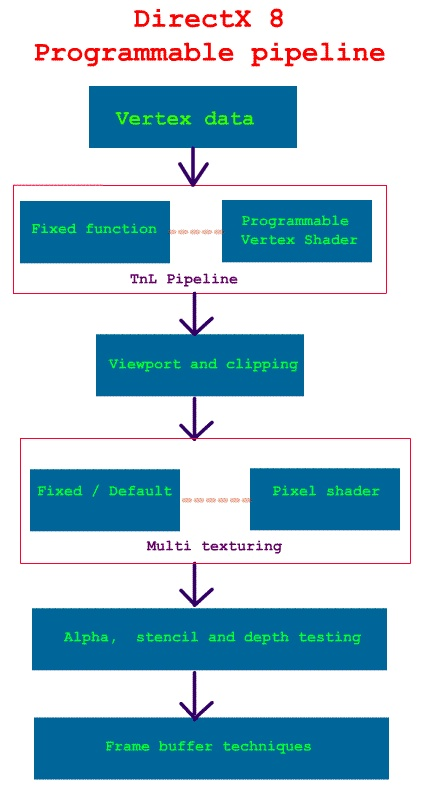What can you do with the vertex shader? Transformations. Lighting model. Geometry skinning. Generate texture co-ordinates on the fly. What you cannot do with a vertex shader? Cannot create new vertex. Cannot do any polygon level calculations, since we are unaware of the other vertices. Backface culling. Cannot modify any vertex data other than itself.

 Architecture Of Vertex Shaders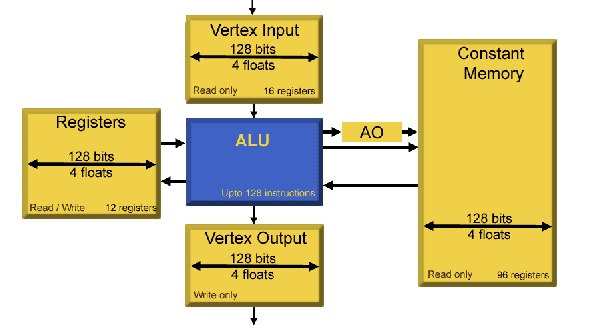Registers Number of registers Properties Input (V0 � V15) 16 x (4 components each) Read only Output 9 x (4 components each) Write only Constants (C0 � C95) 96 x (4 components each) Read only Temporary (R0 � R11) 12 x (4 components each) Read / Write Address (Only after version 1.1) 1 x (1 component) Read / Write

The numbers of registers are not going to remain the same. In fact the table given above holds good only for the current generation of video cards like GEForce3. You can query the number of available vertex shader constant registers by using the function GetDeviceCaps.

Each register can hold upto 4 floats, i.e, 128 bits.
Visualize each register as having x, y, z and w components.Registers and their names:
• Constant: C0 to C95.
• Input: V0 to V15.
• Output:
oPos = Position in clip space
oD0 = Color 1 (Diffuse color r,g,b,a in the range of 0.0f to 1.0f)
oD1 = Color 2 (Specular color r,g,b,a in the range of 0.0f to 1.0f)
oFog = Fog
oPts = Point size
oT0 to oT3 = Upto 4 texture co-ordinates. One for each hardware texture unit.
• Temporary: R0 to R11
• Address: A0. Only x component can be used.

• GPU uses SIMD (Single Instruction Multiple Data) technology.

All operations are done on the GPU when using HARDWARE_VERTEXPROCESSING. If using SOFTWARE_VERTEXPROCESSING, then the GPU is simulated on the CPU by DirectX using processor specific optimizations. Obviously, it's not as fast as the GPU. If you are debugging your vertex shader code, then, it is a must that you use software vertex processing.

• GPU is faster than the fastest CPU.
• Watch SIMD devil at it's very best.
• Vertex shader code usually sits on the card. Hence, not too much time lost between shader switches.
• Almost all instructions take only one GPU cycle to execute.
• Lot of registers available to play around.
• Operations are done on a per-vertex basis.

What happens to the vertex data after it leaves the vertex shader?
• Clipping.
• Backface cull.
• Perspective division (Homogenous divide).
• Viewport transform.
• Rasterizer.

•  About The Vertex Shader Instruction Set And AssemblerInstructions available:

Click on any of the instructions to see their details.

Click on any of the instructions to see their details.

 Instruction Expansion (in English) Execution time in GPU cycles. � nop No operation 1 � mov Move 1 � add Add 1 � mul Multiply 1 � mad Multiply and add. 1 � dp3 Computes the dotproduct with 3 components. 1 � dp4 Computes the dotproduct with 4 components. 1 � dst Distance attenuation. 1 � lit Computes lighting coefficients from two dot products and a power. 1 � min Minimum. 1 � max Maximum. 1 � slt Set if less than. 1 � sge Set if greater than or equal to. 1 � expp Exponential 10-bit. 1 � log Logarithm 10-bit precision 1 � rcp Reciprocal (1.0f / number) > 1 � rsq Reciprocal of square root. (1/sqrt(num)) > 1

Conventions:
• First sentence expands the instruction in English.
• Second sentence is the instruction format (in bold and italic).
• Instruction format is OpCode followed by Operands.
Like Intel format, destination (register) is always preceded by the Opcode.
• Any sentence after this explains the instruction in more detail.
• All opcodes and operands are in italic.
• �n� in an operand means any one of x, y, z, and w.
nop: No operation
nop

Used as a place holder.

mov: Move
mov dest, src

mul: Multiply
mul dest, src1, src2

Multiply the contents of src1 and src2 and put the result into dest.

src1and src2 can also be dest.

dest = (src1 * src2) + src3

dest = src1 + src2

rsq: Reciprocal of square root. (1/sqrt(num))
rsq dest, src.n

�n� has to be one of x, y, z, and w. if n is not specified, then, it is assumed to be w. Calculates the reciprocal of square root of a number. Used extensively in calculating distance, normalizing vectors, etc.

dp3: Computes the dotproduct with 3 components.
dp3 dest, src1, src2

dest.x = dest.y = dest.z = dest.w =
(src1.x*src2.x) +
(src1.y*src2.y) +
(src1.z*src2.z)

dp4: Computes the dotproduct with 4 components.
dp4 dest, src1, src2

dest.x = dest.y = dest.z = dest.w =
(src1.x*src2.x) +
(src1.y*src2.y) +
(src1.z*src2.z) +
(src1.w*src2.w)

Since dotproducts return scalar value, you would normally use something like this: dp4 dest.n, src1, src2

dst: Distance attenuation.
dst dest, src1.n, src2.n

This sets dest to (1.0f, d, d*d, 1/d). Does this look like what is required for the distance attenuation calculation. Well, exactly.

lit: Computes lighting coefficients from two dot products and power.
lit dest, src
src.x= n dot l
src.y= n dot h
src.zis unused
src.w= p

where,

l= light normal.
h= half angle vector.
n= normal.

P= Power (range +128 to �128)

dest is filled with (1.0f, src. x, L, 1.0f)

min: Minimum
min dest, src1, src2

Sets the destination to the lower of the values in the two source registers.
Ex. min r1, r10, c2 sets the x,y,z, and w components of r1 with the component-wise minimum values from r10 and c2. This is similar to writing min r1.xyzw, r10.xyzw, c2.xyzw

max: Maximum
max dest, src1, src2

Sets the destination to the higher of the values in the two source registers.

(This instruction operates componentwise.)

slt: Set if less than (component-wise).
slt dest, src1, src2

This translates to dest = (src1
sge: Set if greater than or equal to (component-wise).
slt dest, src1, src2

This translates to dest= (src1 >= src2) ? 1 : 0 in C.

expp: Exponential 10-bit precision
expp dest, src. W

The components of the destination register is filled in this manner :

dest.x = 2 ** (int) src. w

dest. y = fractional part (src. w)

dest. z = 2 ** src. w

dest. w = 1.0

log: Logarithm 10-bit precision
log dest, src. W

The components of the destination register is filled in this manner :

dest.x = exponent((int) src. w)

dest.y = mantissa(src. w)

dest.z = log2(src. w)

dest.w = 1.0

rcp: Reciprocal of a number.
rcp dest, src

Allows us to do division.

Limitations of some instructions:
Some instructions have limitations on how the registers can be used.

Ex. add r0 , c4, c3

will give an error that the maximum number of constant registers add can read is one.

But this line will not give an error: add r0 , r4, r3

The above instruction means ->

r0.x = -c4.x + c2.x
r0.y = -c4.y + c2.y
r0.z = -c4.z + c2.z
r0.w = -c4.w + c2.w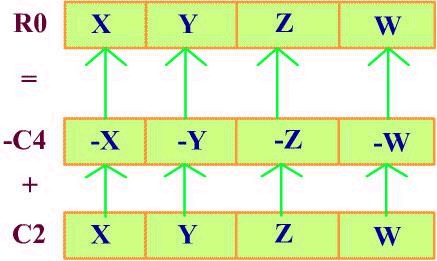Do not use:

mov r2, -c4

You can swizzle the components of the register.
Ex. add r0 , c4.yxzy, r3.xywz
The above instruction means ->

r0.x = c4.y + r3.x
r0.y = c4.x + r3.y
r0.z = c4.z + r3.w
r0.w = c4.y + r3.zDestination register can mask which components are written to.

R1 . . . . write all components
R1. x . . . . write only x component
R1. xw . . . . write only x, w components

For assembling a vertex shader, you will need the vertex shader assembly source and its declaration. The declaration describes the (flexible) vertex format.

You can create your vertex shader in two ways (that I know).

1. Write the assembler as a string with each complete instruction terminated by a new line character. Use the standard DirectX function to assemble the shader and to create the shader.

This is pretty rigid and does not support macros.

2. Write the assembler code in a text file and assemble using the NVidia� assembler (available on the NVidia web site).

As you see, this is very flexible and supports macros.

Its usage format is nvasm.exe �x Input.vsi Input.vso

• You have instructions (damn good ones) to perform all the TnL vertex operations.
• Instructions tailor made for vector, matrix and lighting math.
• If some instructions are missing, then you can achieve it through the existing instructions. Example. Divide = (reciprocal and multiply)
• Up to four data (components) can be manipulated simultaneously with a single instruction.
• Up to 128 instructions can be encoded in a single vertex shader.
• No conditional instructions. They have been sacrificed for speed and performance.
• Take advantage of the free negation and swizzling.

Tips on writing good Vertex shader code:
• Pause and think before using a mov instruction. Much more can be done by other complex instructions and at the same cost.
• Vectorize operations wherever you can.
• The performance of your code is directly dependant on the number of instructions in your shader.
t = (Number of instructions) / (GPU Clock frequency)
• Make use of complex instructions like mad, dst, lit, etc. wherever possible.
• Try to keep data in multiples of four. Like four lights, four blending weights, etc.
• While setting vertex shader constant data, try to set all the data in one SetVertexShaderConstant function rather than multiple calls.
• Try to batch drawprimitive calls in such a way that some common constants need not be set again and again.
• If you are doing some calculation which is per object rather than per vertex, then do it on the CPU and upload it to the vertex shader as a constant, rather than doing it on the GPU.

Ex. (World x View x Projection) matrix.

•  Introduction To DirectX Lighting ModelIn this section, we shall see how surfaces are shaded based on position, orientation, and characteristics of the surfaces and the light sources illuminating them.

First, let's begin by discussing different kinds of light sources (of course, the only source of light for us geeks is the monitor) that are in practice today.

This is in increasing order of computation complexity.
• 1) Ambient light: As the name suggests, this light is present everywhere. This light is a result of light rays being reflected multiple numbers of times by many objects in the ambience. We can never determine the exact source of light. Since this light is present everywhere, it affects all the surfaces with the same intensity.
• 2) Directional light: This light has only direction (orientation) and color. A very good example of directional light is sun light. The source of a directional light is assumed to be at infinite distance from the object / surface.
• 3) Point light: This light has position and color. A good example of point light is your light bulb. Rays from the point light eject uniformly in all directions from its origin.

Depending on the distance and direction to the light source, the object's brightness changes.
• 4) Spotlight: A light source that emits a cone of light. Only objects within the cone are illuminated. The cone produces light of two degrees of intensity, with a central brightly lit section that acts as a point source, and a surrounding dimly lit section
•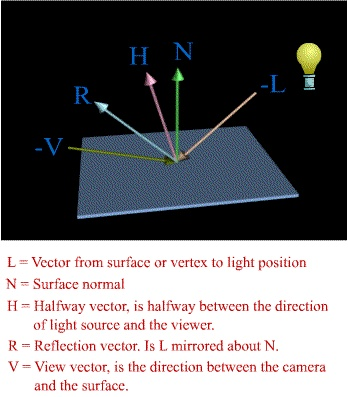We will be discussing only point lights and the diffuse lighting model in this document.

According to Lambert's law, the amount of light seen by the viewer is independent of the viewer's direction and is proportional only to cos q, the angle of incidence of light.

Surfaces that exhibit diffuse reflection or Lambertian reflection appear equally bright from all viewing angles because they reflect light with equal intensity in all directions.

For a given surface, the brightness depends only on the angle q between the direction to the light source L and the surface normal N.Since, point lights have position also, the distance from the surface to the light also affects the intensity. This is known as distance attenuation.

Light Attenuation for point lights
Typically, the energy from the point light that hits a surface diminishes as the distance increases.

 ``` 1 fatt = --------- dL```

Practically, inverse linear falloff doesn't look good. The inverse squared falloff looks better.

 ``` 1 fatt = --------- dL . dL```

In practice, this also doesn't look good. If light is far away, then its intensity is too low. But, when it is close up to the surface, intensity becomes large leading to visual artifacts.

A decent attenuation equation which can be used to get wider range of effects is:

 ``` 1fatt = -------------------------- A0 + A1.dL + A2.dL.dL```

In the above equation, A0, A1 and A2 are known as the attenuation constants.

As d goes to infinity, attenuation becomes very small but not zero.

By varying the three constants A0, A1, A2, interesting effects can be achieved.
• Radial distance falloff: A1 = 0 and A2 > 0
• Linear falloff: A1 > 0 and A2 = 0
• If the light is too close to the surface, then, attenuation becomes very large, making the light too bright. To avoid this, set A0 to 1 or greater.

All light colors are represented in ranges from (0.0 to 1.0), where 0 = least intensity and 1 is the maximum intensity.

Material Properties
Every surface has a material property. Of course, the material property can be global to all the surfaces. There are different kinds of material properties like diffuse, specular, ambient, etc. The ambient and diffuse material properties determine how much light the surface reflects. Ex., If a surface has a diffuse material property of (1,0,0) corresponding to the red, green and blue components of the light respectively, then this surface reflects only the red component whereas the green and blue components will be absorbed.

Lighting Equation
Even with the attenuation factor blending into our lighting equation, still, the maximum amount of light a surface receives is (N.L).

The final diffuse lighting equation for becomes:Vertex lighting V/S Polygonal lighting
Polygonal lighting: Light intensity is calculated for each surface / polygon.
Vertex lighting: Light intensity is calculated for every vertex. The intensities will be interpolated linearly across the polygon by the Gouraud shader.Vertex BlendingLet's take some time off to take a quick look at the different kinds of animation systems in practice today.
• Vertex based animation:
This is the most primitive of the animation systems. Vertex positions (may include normals also) for all the vertices in the mesh for all the animation frames are stored in memory.

This is the fastest way of animating, but its memory bandwidth is too high.

It is also visually not appealing, since animation frames are jumped integrally. The intermediate positions in between animation frames are skipped totally.

Also, it needs extra transition animations to switch between animations.

Ex. Visualize that a character is doing a walk animation. Say, its animation is changed to sit. It would look too shabby if the animation was switched abruptly to sit from walk.

So, first, an intermediate animation has to be played, and then, from intermediate to the target animation.

Memory requirement of the mesh multiplies with each added animation.

 ``` Ex.: Current animation Intermediate Target animation Walk (loop) -> Stand -> Sit ```

• Vertex based key-frame animation:
This is a slight improvement over the vertex based animation. The only difference is that, the vertex positions are stored only for key-frames. The intermediate vertex positions are computed dynamically using interpolation schemes such as linear, Hermite curve, etc.

And also, the number of key frames can be slightly lesser than what it would have been with pure vertex based animation.

Visually, it is slightly more appealing than pure vertex based animation, since the intermediate frames are filled in dynamically at run-time.

Transition frames are not needed. Even transition is achieved by interpolation.

• Skeletal animation system:
3D game developers are realizing that skeletal animation system is very versatile for both programmers as well as artists (actually more for the artists).

Skeletal animation system consists of a series of bones which are hierarchical in nature.

Mesh (vertices) or skin is attached to the bones. Hence, when the bones move/rotate, the vertices attached to the bone also move/rotate.

Only the bone data needs to be stored for every frame of the animation. Usually, the bone data is represented by quaternions.

This eliminates the need to store vertex positions for all the vertices for every frame of the animation, as in the vertex based animation.

• Smooth animation. We can derive intermediate frames by using spherical interpolation.
• Smooth transition while changing from one animation to the other.
• Memory bandwidth is very small.
• Any number of animations can be added whereas the mesh remains constant.

• Let's take a look at what we discussed above, in figures.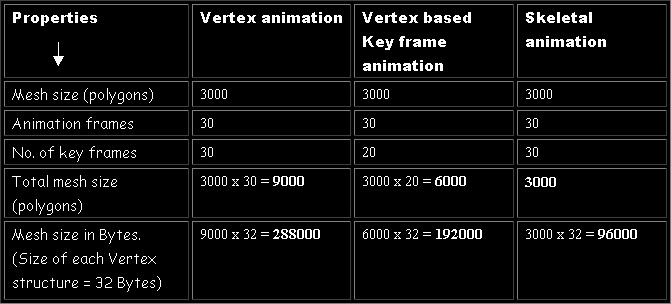The skeletal animation system looks perfect doesn't it?
Well, nothing is perfect in 3D Games. Skeletal animation comes with its own set of problems.

Let's study about the drawbacks of skeletal animation system with the help of some images.

The following image shows the relationship of the skeleton / bone with its mesh. The semi-transparent object is the mesh. As you see, they are tightly coupled. When a bone moves, the vertices attached to it also move.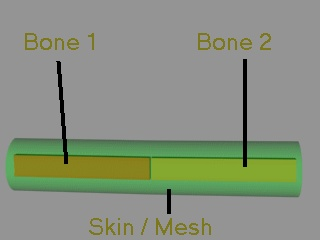The following image shows the same relationship as explained above. The only difference is that the mesh is shown in wire frame.

The green line divides the vertices between the first and second bone. Vertices are represented by a small "+" sign. Vertices on the right are marked red indicating that they belong to the second bone, where as the vertices on the left are marked white indicating that they belong to the first bone.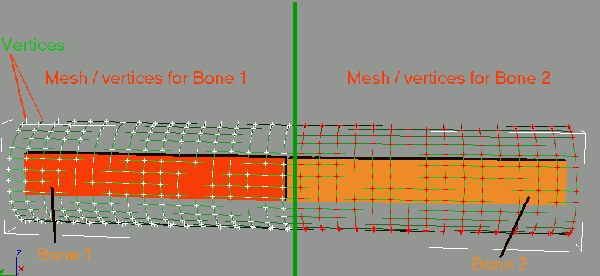The following figure highlights the main drawback of the skeletal animation system.The following figure shows how the "stiffness" problem can be solved. A quantum leap isn't it? No dinner comes free. Likewise, vertex blending is not free. It adds to computational cost.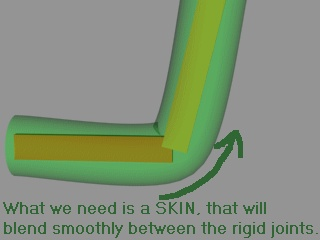Animation with vertex blending:Animation without vertex blending: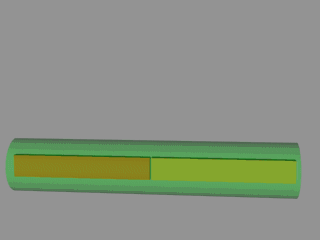Everything is moving smoothly with vertex blending. Let's move to the rough part - the math behind vertex blending. Surprisingly, the math is very simple, probably too simple.

The generic blending formula: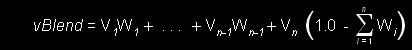where,
vBlend = output vertex.
Vn = nth vertex.
Wn = nth vertex's weight.

For two, three and four weighted matrices, the above formula becomes:Consider an example:
 ``` V' = Final blended position. V = VertexPosition M1 = WorldMatrix 1 M2 = WorldMatrix 2 M3 = WorldMatrix 3 W1 = Weight1, W2 = Weight2 V' = (W1)( V * M1 ) + (W2)( V * M2 ) + (1- W2 - W1)(V*M3)Let's see how we can do vertex blending for two weights. for (every vertex of the mesh) do { V = current vertex. M [] = array of bone matrices. id0 = First index in to the bone matrix array. id1 = Second index in to the bone matrix array. W0 = Weight corresponding to index id0. W1 = Weight corresponding to index id1. W0 and W1 are normalized. i.e. W0+W1 = 1 V' = ( ( V x M[id0] ) x W0 ) + ( ( V x M[id1] ) x W1 ) } ```

Typically, the vertex structure for a blending vertex looks like this: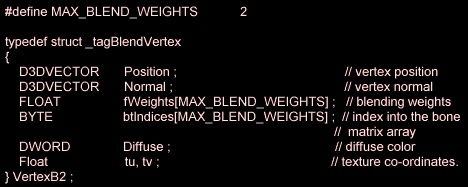How can transformation, lighting and blending be achieved using the vertex shader?
Till now we have only talked about vertex shaders and lighting models. Let's take a look at how they actually work.

Before we start off solving the problem, we need to look at the resources that we need. I have decided to use a tesselated plane as my surface. And I have a texture that I can apply on the plane. We shall set up the plane, keeping in mind, that we also have to blend (skin) later.

We do not want to come back to setting up the plane again. So we will first finish the complete initialization (position, weights, indices and texture co-ordinates) of the plane and then deal with the problem one by one. About the lights, I am using two positional (point) lights. I am assuming that the surface material has a diffuse reflection property of (1,1,1). i.e., the surface reflects all three colors with the same intensity.

See Listing 1 for all the global variables, macros and structure declaration.

The plane itself is tesselated to a certain level. This is controlled by the two macros

 ``` #define MAX_PLANE_TESS_LEVEL_HORZ 3 // horizontal tesselation level #define MAX_PLANE_TESS_LEVEL_VERT 3 // vertical tesselation level ```

Of course, the plane has a certain width and height. It is controlled by the following macros

 ``` #define PLANE_WIDTH 40 // physical width of the plane #define PLANE_HEIGHT 30 // physical height of the plane ```

The plane is actually built in the way as described in the figure below. The pivot is at the origin for both parts of the plane. If the pivot is not at the origin, then some extra work has to be done before rotating the planes.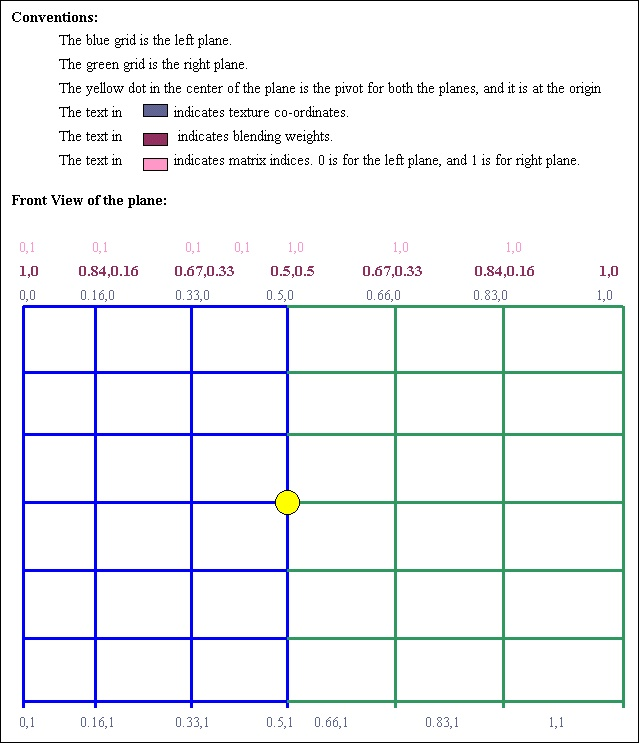See Listing 2 which has the source code for the above explaination. You will find some utility functions like InitVertexBuffer and LoadTexture in the Sources Zip file.

1.4.0f, 22.0f, 1.0f, 0.0f on to C0
2.Four zeroes into C1.
3.Transposed world matrix from C14 to C18.
4.Transposed (View x Projection) matrix from C18 to C21.
5.Light 0 Position into C2.
6.Light 0 Attenuation into C3.
7.Light 0 Color into C4.
8.Light 1 Position into C5.
9.Light 1 Attenuation into C6.
10.Light 1 Color into C7.
11.Transposed rotation matrix for the left plane from C22 to C25.
12.Transposed rotation matrix for the right plane from C26 to C29. Why so many constants are loaded will be explained as and when they are required.

Now we have the plane data ready to be displayed. Let's look at the code which sets up the vertex shader constants and calls drawpprimitive.

The matrix data should be transposed before loading into the vertex shader. This is because, the vertex shader operates on row basis and our DirectX matrices operate on column basis.

Note that only the final matrix should be transposed. I.e, transpose the result of (View x Projection) matrix instead of transposing view and projection matrices seperately and multiplying them (which is wrong). This is explained below.dp4 is used to multiply the vertex position with the world matrix.
dp4 operates only components of a single register.
It does on operate on C14.x, C15.x, C16.x and C17.x simultaneously. But it operates on C14.x, C14.y, C14.z, C14.w simultaneously. Hence we need to transpose the matrix.

Now it becomes,Also note that (View x Projection) matrix is per scene, not per vertex. Hence they are multiplied on the CPU just once per scene (GameLoop). The world (transposed) matrix is loaded seperately, since we need this for lighting calculations.

Since I am using NVidia� assembler, I can use macros. I have defined some macros for loading some common constants.

See Listing 3 for the common constants declaration.

1. Transforming the vertex:
First, let's start off by just transforming the plane vertices into clip space and setting their texture co-ordinates.

For our first solution, we need only two constants, i.e world matrix and the (view x projection) matrices (both transposed). You can ignore the loading of other constants for now. See Listing 4, which has the vertex shader to do just this.

This is the simplest of the shaders. I'm doing just this:
• a. CLIP Position = (Vertex) x (World Matrix) x (View x projection)
• b. Set all color components to 1(i.e full intensity).
• c. Copy the texture co-ordinates.

• 2. Lighting the vertex:
This involves some extra work for lighting the vertices. For this, we need the light properties which are loaded into the appropriate vertex shader constant registers.

See Listing 5, which has the vertex shader to do just this.

I have used a macro NORMALIZE to normalize a vector. It accepts two vectors as parameters. This shader does the following things:
• a.World Vertex Position = (Vertex) x (World Matrix)
• b.Position in CLIP space for the vertex = World Vertex Position x (View x projection)
• c.Transform the normal into world co-ordinates.
• d.Calculate the intensity at the vertex for all the given lights. Here I'm using only two point lights.
• e.Calculate the final intensity as a result of all the lights affecting this vertex.
• f.Copy texture co-ordinates.
• Lighting is calculated in the following manner inside the shader:

R0 has the transformed vertex normal.
R9 has the vertex position in world co-ordinates.
C2 has light 0's position.
C3 has light 0's attenuation.
C4 has light 0's color.

First, calculate the vector between the vertex in it's world position and the light position.
Direction vector (R10) = C2 � R9

Normalize R10 such that R11.xyz holds the normalized vector and R10.w holds the squared distance (d*d) between the light and the vertex.
R11.w = linear distance (d) between the light and the vertex.

Find the cosine of the angle between the vertex normal and the newly calculated vertex to light normal.

 `dp3 r6.x, r11, r0 ; N.L `

Setup the attenuation equation in R4.

 `dst r4, r10.w, r11.w ; (1, d, d*d, 1/d) `

Calculate fatt in R5.x
 ``` dp3 r5.x, r4, c[CV_LIGHT0_ATT] ; (a0 + a1*d + a2*d*d) rcp r5.x, r5.x ; 1 / (a0 + a1*d + a2*d*d) ```

Similarly calculate the attenuation fatt for the other lights (upto four) and put them in R5.y, R5.z and R5.w respectively.
Put the (N.L) for the other three lights in R6.y, R6.z and R6.w respectively.

Now R5 has attenuation for the four lights respectively. R6 has the intensity for the four lights respectively. However, in this sample, only two lights are being used. Hence, only the x any y components are valid.

Now you can vectorize light calculations (for two lights) in this way.

 ```max r6.xy, r6.xy, CV_ZERO ; max(N.L, 0.0f) mul r7, r6.xy, r5.xy ; factor = max(N.L, 0.0f) * attenuation mul r8.xyz, c[CV_LIGHT0_COLOR].xyz, r7.x ; vertex_color = light_color * factor mad oD0.xyz, c[CV_LIGHT1_COLOR].xyz, r7.y, r8.xyz ; add other lights ```

3. Vertex Blending:
Lets take a look at how blending can be achieved on the vertex shader.

The following three lines from the vertex shader code determine the absolute offset into the matrix array which is stored as constants.

 ``` add r0, V_INDICES, -CV_BONE_OFFSET mad r0, r0, CV_EACH_BONEMAT_HEIGHT, V_BONE_MAT_START_INDEX max r0, r0, CV_ZERO ```

r0 will hold the absolute offsets into the four blending weights. Of course, here we are using only two. The rest of the code does implement the blending equation.

4. Mesh without blending:
This vertex shader just transforms the plane by applying their respective rotations. It neither blends nor lights it.

5. Blending, with light:
This vertex shader blends the mesh and also lights it. For lighting, even the vertex normals have to be blended.

 Closing